# High School Physics : Representations of Motion

## Example Questions

### Example Question #1 : Representations Of Motion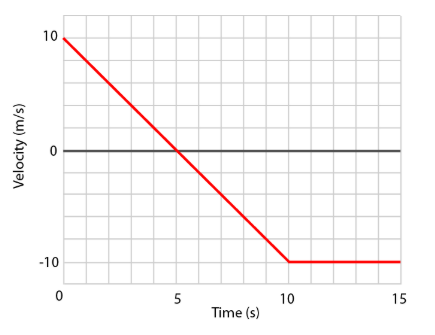Calculate the total displacement of the object.

Possible Answers: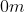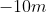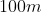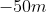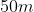Correct answer:Explanation:

This is a velocity versus time graph.  The displacement can be determined from the area under the curve of the graph.

Begin with the first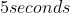.  This is a triangle with a base of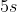and height of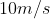.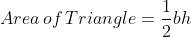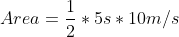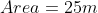Next look at the next.  This is a triangle with a base ofand height of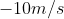.  That negative is important as it indicates that the object was traveling in the opposite direction.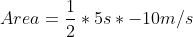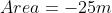For the final, the area is that of a rectangle with a base ofand a height of. Again this negative is important as it indicates what direction the object was traveling in.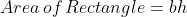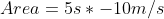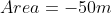To find the total displacement, add all of the areas together.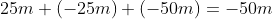Therefore the final displacement is.

### Example Question #2 : Representations Of Motion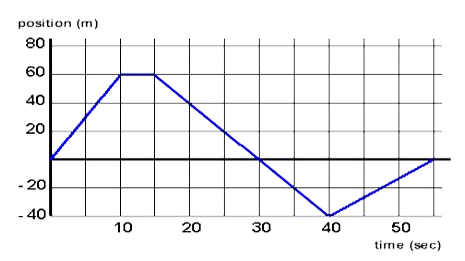What is the object’s total distance traveled at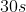?  What is the object’s displacement at?

Possible Answers: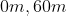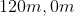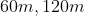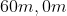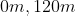Correct answer:Explanation:

This is a position versus time graph.  The total distance traveled is the total position that has been traveled during the entire time.

From 0 to 10 seconds, the object traveled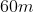.

From 10 seconds to 15 seconds, the object did not move.

From 15 seconds to 30 seconds the object traveledbackward.

The distance is the total amount traveled.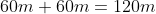The displacement which is the distance traveled from the original position.  Since at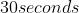the object has returned to the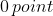, the displacement is.

### Example Question #3 : Representations Of MotionWhat is the acceleration of the object at?

Possible Answers: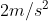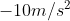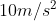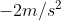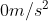Correct answer:Explanation:

This is a position versus time graph.  To find the velocity of an object at a particular time, determine the slope of the graph at that time.  Using the points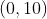and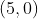we can determine the slope of the graph at 5 seconds since it is a constant slope.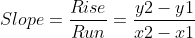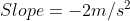### Example Question #4 : Representations Of Motion

You are given a graph of displacement vs. time. Which of the following ways can be used to determine velocity at any given point?

Possible Answers:

The velocity is the y-intercept of the graph

The velocity is the area under the curve for any time interval

The velocity is the slope of the line at any given time

The velocity is the total change in displacement over the total time

The velocity cannot be determined from a graph of displacement vs time

Correct answer:

The velocity is the slope of the line at any given time

Explanation:

The important thing to note is that the question asks for the velocity at any given point. The average velocity will be equal to the total displacement divided by the total time, but the question is asking for the instantaneous velocity.

Velocity is calculated by a change in displacement over a change in time. In a displacement versus time graph, this is equal to the slope.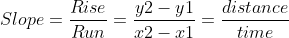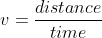This tells us that the slope at a certain time will be equal to the velocity at that time.

In calculus terms, velocity is the derivative of the function for displacement in terms of time. What this means is that at any point on the graph, the instantaneous slope is the velocity for that given time. To determine velocity, one must find the slope of the line at that particular time interval.

### Example Question #5 : Representations Of Motion

You are given a graph of velocity vs. time. Which of the following ways can be used to determine acceleration at any given point?

Possible Answers:

The acceleration is the area under the curve for any given time interval

The acceleration is the y-intercept of the velocity vs time graph

The acceleration is the total change in velocity over the total time

The acceleration cannot be determined from the graph

The acceleration is the slope at any particular point in time

Correct answer:

The acceleration is the slope at any particular point in time

Explanation:

The important thing to note is that the question asks for the acceleration at any given point. The average acceleration will be equal to the net change in velocity divided by the total time, but the question is asking for the instantaneous acceleration.

Acceleration is calculated by a change in velocity over a change in time. In a velocity versus time graph, this is equal to the slope.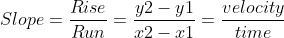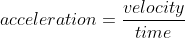This tells us that the slope at a certain time will be equal to the acceleration at that time.

In calculus terms, acceleration is the derivative of the function for velocity in terms of time. What this means is that at any point on the graph, the instantaneous slope is the acceleration for that given time. To determine acceleration, one must find the slope of the line at that particular time interval.

### Example Question #6 : Representations Of Motion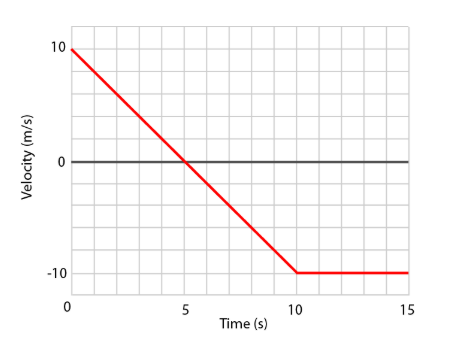Describe the motion of the object.

Possible Answers:

The object is slowing down for the first 5 seconds.  Then the object is speeding up for the next 5 seconds.  Then the object travels at a constant velocity.

The object travels with a negative velocity for 10 seconds and then travels at a constant velocity.

The object is slowing down for 10 seconds.  Then the object travels at a constant speed.

The object travels in the positive direction for 5 seconds.  Then the object travels in the negative direction at the same speed for the next 5 seconds.  Then the object stops moving.

The object travels in the negative direction for 10 seconds and then does not move.

Correct answer:

The object is slowing down for the first 5 seconds.  Then the object is speeding up for the next 5 seconds.  Then the object travels at a constant velocity.

Explanation:

This is a velocity versus time graph.  During the first 5 seconds, the velocity is approaching 0.  This means that the object is slowing down.

During the next 5 seconds, the velocity is increasing in the negative direction.  This means that the object is speeding up in the negative direction.

During the final 5 seconds, the velocity value does not change.  This means that the object is remaining at a constant velocity.

### Example Question #7 : Representations Of MotionWhat is the velocity of the object at 25 s?

Possible Answers: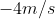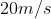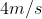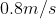Correct answer:Explanation:

This is a position versus time graph.  To find the velocity of an object at a particular time, determine the slope of the graph at that time.  Using the points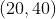and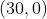we can determine the slope of the graph at 25 seconds since it is a constant slope.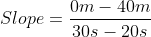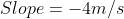### Example Question #361 : Motion And Mechanics

You are given a graph of velocity vs. time. How can you find the change in displacement for a particular time interval?

Possible Answers:

The displacement cannot be determined from the graph

The displacement is the average slope during the given time interval

The displacement can only be found when the velocity crosses the x-axis

The displacement is the area under the curve for the given time interval

The displacement is the total area under the curve of the graph

Correct answer:

The displacement is the area under the curve for the given time interval

Explanation:

Velocity is equal to a change in displacement per unit time. Consider a basic velocity vs. time graph that depicts a constant velocity, given by a straight horizontal line. How can we determine the displacement during a given time interval? Use the kinematics equation: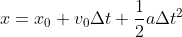Acceleration will be zero, allowing us to reduce the equation. We can also assume that there is zero initial displacement.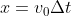So, the displacement will be equal to the velocity multiplied by the time. In the graph, velocity will be represented by the vertical location and time will be represented by the horizontal location of any point. Displacement will be equal to the height of the graph times the width of the graph, or the area of the rectangle created by the constant velocity over a unit of time.

Though this is just one example, displacement will always be equal to the area under the curve of a velocity vs. time graph.

In calculus terms, velocity is the derivative of the function for displacement in terms of time. To find the displacement, one must take the integral of the velocity function. The result is the area under the curve of the velocity function.

### Example Question #9 : Representations Of MotionDescribe the motion of the object.

Possible Answers:

The object travels at a constant speed for the first 10 seconds.  Then the object does not move.  At 15 seconds it travels at a constant speed in the opposite direction.  At 40 seconds the object turns around again and travels at a constant speed.

The object speeds up for 10 seconds, then remains at a constant speed.  At 15 seconds it starts to slow down until 40 seconds.  Then it speeds up for the final 20 seconds.

The object speeds up for the first 10 seconds.  Then the object remains at a constant speed.  Then the object travels in the positive direction with a positive speed until 30 seconds.  At 30 seconds it travels in the negative direction with a negative speed for the remaining time.

The object travels at a constant speed, then does not move, then slows down until 40s.  Then the object speeds up for the final 20 seconds.

Correct answer:

The object travels at a constant speed for the first 10 seconds.  Then the object does not move.  At 15 seconds it travels at a constant speed in the opposite direction.  At 40 seconds the object turns around again and travels at a constant speed.

Explanation:

This is a position versus time graph.  The slope of the line of the graph tells us the velocity of the object.

For the first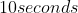the object has a constant positive slope indicating that the object is traveling at a constant positive speed.

From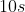to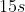the object remains at the same position which means that the object has stopped.

Fromto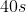the object has a constant negative slope meaning that the object is traveling at a constant negative speed.

At, the object switches directions and now has a constant positive slope meaning that the object is traveling at a constant positive speed.

### All High School Physics Resources